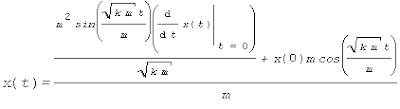## Tuesday, February 27, 2007

### Balbir's log - 27 Feb 2007

1. Played around with Scilab, used scilab for some simple signal processing work, Amplitude Modulation.
2. I've started reading a paper on VM entitled Making LRU friendly to weak locality workloads
3. Added Markov chains to my to-learn list

## Saturday, February 24, 2007

### Using maxima -- baby steps

The use of symbolic computing makes it easy to visualize and solve problems that were otherwise dry and would put you to sleep. To test the simplicity of a freely available tool called maxima, I tried to solve a common problem with it.

The problem is quite simple, remember high school physics? Well I don't :-). Consider a tuning fork, which oscillates and produces a sound (depending on it's frequency).

We start by entering the mathematical model of the fork

'm*diff(x(t), t, 2)=-k*x(t);

Maxima printsWe now request maxima to solve the equation for us by

desolve([%],[x(t)]);

Is k * m positive, negative, or zero? We say positive

Maxima printsWe ask maxima to simplify the result for us